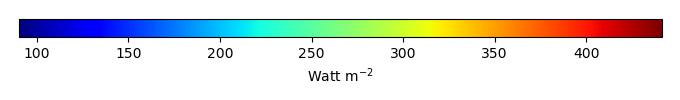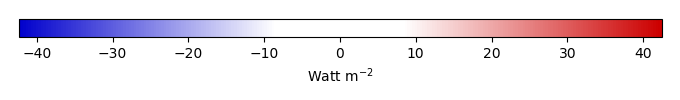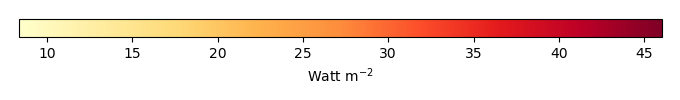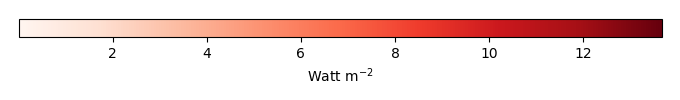# Mean State

Period Mean (original grids) [Watt m-2]
Model Period Mean (intersection) [Watt m-2]
Model Period Mean (complement) [Watt m-2]
Benchmark Period Mean (intersection) [Watt m-2]
Benchmark Period Mean (complement) [Watt m-2]
Bias [Watt m-2]
RMSE [Watt m-2]
Phase Shift [months]
Bias Score 
RMSE Score 
Seasonal Cycle Score 
Spatial Distribution Score 
Interannual Variability Score 
Overall Score 
Benchmark [-] 397.
CLM4 [-] 406. 406. 396. 408. 11.3 16.6 0.567 0.327 0.403 0.940 0.763 0.761 0.599
CLM4.5 [-] 406. 406. 396. 408. 11.3 16.6 0.567 0.327 0.403 0.940 0.763 0.761 0.599
CLM5 [-] 406. 406. 396. 408. 11.3 16.6 0.567 0.327 0.403 0.940 0.763 0.761 0.599
Period Mean (original grids) [Watt m-2]
Model Period Mean (intersection) [Watt m-2]
Model Period Mean (complement) [Watt m-2]
Benchmark Period Mean (intersection) [Watt m-2]
Benchmark Period Mean (complement) [Watt m-2]
Bias [Watt m-2]
RMSE [Watt m-2]
Phase Shift [months]
Bias Score 
RMSE Score 
Seasonal Cycle Score 
Spatial Distribution Score 
Interannual Variability Score 
Overall Score 
Benchmark [-] 384.
CLM4 [-] 395. 394. 384. 397. 10.5 17.7 0.930 0.614 0.634 0.889 0.831 0.742 0.724
CLM4.5 [-] 395. 394. 384. 397. 10.5 17.7 0.930 0.614 0.634 0.889 0.831 0.742 0.724
CLM5 [-] 395. 394. 384. 397. 10.5 17.7 0.930 0.614 0.634 0.889 0.831 0.742 0.724
Period Mean (original grids) [Watt m-2]
Model Period Mean (intersection) [Watt m-2]
Model Period Mean (complement) [Watt m-2]
Benchmark Period Mean (intersection) [Watt m-2]
Benchmark Period Mean (complement) [Watt m-2]
Bias [Watt m-2]
RMSE [Watt m-2]
Phase Shift [months]
Bias Score 
RMSE Score 
Seasonal Cycle Score 
Spatial Distribution Score 
Interannual Variability Score 
Overall Score 
Benchmark [-] 362.
CLM4 [-] 369. 368. 361. 382. 9.69 17.7 1.20 0.620 0.625 0.840 0.968 0.714 0.732
CLM4.5 [-] 369. 368. 361. 382. 9.69 17.7 1.20 0.620 0.625 0.840 0.968 0.714 0.732
CLM5 [-] 369. 368. 361. 382. 9.69 17.7 1.20 0.620 0.625 0.840 0.968 0.714 0.732
Period Mean (original grids) [Watt m-2]
Model Period Mean (intersection) [Watt m-2]
Model Period Mean (complement) [Watt m-2]
Benchmark Period Mean (intersection) [Watt m-2]
Benchmark Period Mean (complement) [Watt m-2]
Bias [Watt m-2]
RMSE [Watt m-2]
Phase Shift [months]
Bias Score 
RMSE Score 
Seasonal Cycle Score 
Spatial Distribution Score 
Interannual Variability Score 
Overall Score 
Benchmark [-] 239.
CLM4 [-] 205. 205. 238. 251. -30.9 33.7 0.0673 0.550 0.783 0.995 0.970 0.740 0.803
CLM4.5 [-] 205. 205. 238. 251. -30.9 33.7 0.0673 0.550 0.783 0.995 0.970 0.740 0.803
CLM5 [-] 205. 205. 238. 251. -30.9 33.7 0.0673 0.550 0.783 0.995 0.970 0.740 0.803
Period Mean (original grids) [Watt m-2]
Model Period Mean (intersection) [Watt m-2]
Model Period Mean (complement) [Watt m-2]
Benchmark Period Mean (intersection) [Watt m-2]
Benchmark Period Mean (complement) [Watt m-2]
Bias [Watt m-2]
RMSE [Watt m-2]
Phase Shift [months]
Bias Score 
RMSE Score 
Seasonal Cycle Score 
Spatial Distribution Score 
Interannual Variability Score 
Overall Score 
Benchmark [-] 411.
CLM4 [-] 427. 427. 410. 415. 19.7 22.9 1.86 0.0696 0.331 0.721 0.566 0.701 0.453
CLM4.5 [-] 427. 427. 410. 415. 19.7 22.9 1.86 0.0696 0.331 0.721 0.566 0.701 0.453
CLM5 [-] 427. 427. 410. 415. 19.7 22.9 1.86 0.0696 0.331 0.721 0.566 0.701 0.453
Period Mean (original grids) [Watt m-2]
Model Period Mean (intersection) [Watt m-2]
Model Period Mean (complement) [Watt m-2]
Benchmark Period Mean (intersection) [Watt m-2]
Benchmark Period Mean (complement) [Watt m-2]
Bias [Watt m-2]
RMSE [Watt m-2]
Phase Shift [months]
Bias Score 
RMSE Score 
Seasonal Cycle Score 
Spatial Distribution Score 
Interannual Variability Score 
Overall Score 
Benchmark [-] 264.
CLM4 [-] 243. 243. 264. 277. -20.2 22.6 0.0306 0.696 0.838 0.998 0.983 0.858 0.869
CLM4.5 [-] 243. 243. 264. 277. -20.2 22.6 0.0306 0.696 0.838 0.998 0.983 0.858 0.869
CLM5 [-] 243. 243. 264. 277. -20.2 22.6 0.0306 0.696 0.838 0.998 0.983 0.858 0.869
Period Mean (original grids) [Watt m-2]
Model Period Mean (intersection) [Watt m-2]
Model Period Mean (complement) [Watt m-2]
Benchmark Period Mean (intersection) [Watt m-2]
Benchmark Period Mean (complement) [Watt m-2]
Bias [Watt m-2]
RMSE [Watt m-2]
Phase Shift [months]
Bias Score 
RMSE Score 
Seasonal Cycle Score 
Spatial Distribution Score 
Interannual Variability Score 
Overall Score 
Benchmark [-] 294.
CLM4 [-] 279. 278. 293. 336. -11.9 19.7 0.0697 0.761 0.775 0.995 0.992 0.827 0.854
CLM4.5 [-] 279. 278. 293. 336. -11.9 19.7 0.0697 0.761 0.775 0.995 0.992 0.827 0.854
CLM5 [-] 279. 278. 293. 336. -11.9 19.7 0.0697 0.761 0.775 0.995 0.992 0.827 0.854
Period Mean (original grids) [Watt m-2]
Model Period Mean (intersection) [Watt m-2]
Model Period Mean (complement) [Watt m-2]
Benchmark Period Mean (intersection) [Watt m-2]
Benchmark Period Mean (complement) [Watt m-2]
Bias [Watt m-2]
RMSE [Watt m-2]
Phase Shift [months]
Bias Score 
RMSE Score 
Seasonal Cycle Score 
Spatial Distribution Score 
Interannual Variability Score 
Overall Score 
Benchmark [-] 379.
CLM4 [-] 385. 385. 377. 410. 14.7 23.2 0.641 0.526 0.667 0.941 0.933 0.740 0.746
CLM4.5 [-] 385. 385. 377. 410. 14.7 23.2 0.641 0.526 0.667 0.941 0.933 0.740 0.746
CLM5 [-] 385. 385. 377. 410. 14.7 23.2 0.641 0.526 0.667 0.941 0.933 0.740 0.746
Period Mean (original grids) [Watt m-2]
Model Period Mean (intersection) [Watt m-2]
Model Period Mean (complement) [Watt m-2]
Benchmark Period Mean (intersection) [Watt m-2]
Benchmark Period Mean (complement) [Watt m-2]
Bias [Watt m-2]
RMSE [Watt m-2]
Phase Shift [months]
Bias Score 
RMSE Score 
Seasonal Cycle Score 
Spatial Distribution Score 
Interannual Variability Score 
Overall Score 
Benchmark [-] 244.
CLM4 [-] 214. 215. 244. 248. -28.8 31.6 0.00253 0.558 0.773 1.00 0.972 0.838 0.819
CLM4.5 [-] 214. 215. 244. 248. -28.8 31.6 0.00253 0.558 0.773 1.00 0.972 0.838 0.819
CLM5 [-] 214. 215. 244. 248. -28.8 31.6 0.00253 0.558 0.773 1.00 0.972 0.838 0.819
Period Mean (original grids) [Watt m-2]
Model Period Mean (intersection) [Watt m-2]
Model Period Mean (complement) [Watt m-2]
Benchmark Period Mean (intersection) [Watt m-2]
Benchmark Period Mean (complement) [Watt m-2]
Bias [Watt m-2]
RMSE [Watt m-2]
Phase Shift [months]
Bias Score 
RMSE Score 
Seasonal Cycle Score 
Spatial Distribution Score 
Interannual Variability Score 
Overall Score 
Benchmark [-] 351.
CLM4 [-] 353. 353. 350. 359. 3.02 14.9 0.381 0.746 0.720 0.974 0.875 0.714 0.792
CLM4.5 [-] 353. 353. 350. 359. 3.02 14.9 0.381 0.746 0.720 0.974 0.875 0.714 0.792
CLM5 [-] 353. 353. 350. 359. 3.02 14.9 0.381 0.746 0.720 0.974 0.875 0.714 0.792
Period Mean (original grids) [Watt m-2]
Model Period Mean (intersection) [Watt m-2]
Model Period Mean (complement) [Watt m-2]
Benchmark Period Mean (intersection) [Watt m-2]
Benchmark Period Mean (complement) [Watt m-2]
Bias [Watt m-2]
RMSE [Watt m-2]
Phase Shift [months]
Bias Score 
RMSE Score 
Seasonal Cycle Score 
Spatial Distribution Score 
Interannual Variability Score 
Overall Score 
Benchmark [-] 308.
CLM4 [-] 290. 290. 307. 324. -13.0 18.2 0.220 0.649 0.738 0.985 0.897 0.856 0.810
CLM4.5 [-] 290. 290. 307. 324. -13.0 18.2 0.220 0.649 0.738 0.985 0.897 0.856 0.810
CLM5 [-] 290. 290. 307. 324. -13.0 18.2 0.220 0.649 0.738 0.985 0.897 0.856 0.810
Period Mean (original grids) [Watt m-2]
Model Period Mean (intersection) [Watt m-2]
Model Period Mean (complement) [Watt m-2]
Benchmark Period Mean (intersection) [Watt m-2]
Benchmark Period Mean (complement) [Watt m-2]
Bias [Watt m-2]
RMSE [Watt m-2]
Phase Shift [months]
Bias Score 
RMSE Score 
Seasonal Cycle Score 
Spatial Distribution Score 
Interannual Variability Score 
Overall Score 
Benchmark [-] 344.
CLM4 [-] 305. 305. 311. 360. -2.78 21.3 0.550 0.591 0.634 0.933 0.991 0.728 0.752
CLM4.5 [-] 305. 305. 311. 360. -2.78 21.3 0.550 0.591 0.634 0.933 0.991 0.728 0.752
CLM5 [-] 305. 305. 311. 360. -2.78 21.3 0.550 0.591 0.634 0.933 0.991 0.728 0.752
Period Mean (original grids) [Watt m-2]
Model Period Mean (intersection) [Watt m-2]
Model Period Mean (complement) [Watt m-2]
Benchmark Period Mean (intersection) [Watt m-2]
Benchmark Period Mean (complement) [Watt m-2]
Bias [Watt m-2]
RMSE [Watt m-2]
Phase Shift [months]
Bias Score 
RMSE Score 
Seasonal Cycle Score 
Spatial Distribution Score 
Interannual Variability Score 
Overall Score 
Benchmark [-] 343.
CLM4 [-] 350. 350. 343. 360. 8.96 20.6 0.463 0.703 0.633 0.956 0.933 0.670 0.755
CLM4.5 [-] 350. 350. 343. 360. 8.96 20.6 0.463 0.703 0.633 0.956 0.933 0.670 0.755
CLM5 [-] 350. 350. 343. 360. 8.96 20.6 0.463 0.703 0.633 0.956 0.933 0.670 0.755
Period Mean (original grids) [Watt m-2]
Model Period Mean (intersection) [Watt m-2]
Model Period Mean (complement) [Watt m-2]
Benchmark Period Mean (intersection) [Watt m-2]
Benchmark Period Mean (complement) [Watt m-2]
Bias [Watt m-2]
RMSE [Watt m-2]
Phase Shift [months]
Bias Score 
RMSE Score 
Seasonal Cycle Score 
Spatial Distribution Score 
Interannual Variability Score 
Overall Score 
Benchmark [-] 244.
CLM4 [-] 216. 216. 243. 253. -27.1 29.3 0.0689 0.559 0.791 0.995 0.989 0.754 0.813
CLM4.5 [-] 216. 216. 243. 253. -27.1 29.3 0.0689 0.559 0.791 0.995 0.989 0.754 0.813
CLM5 [-] 216. 216. 243. 253. -27.1 29.3 0.0689 0.559 0.791 0.995 0.989 0.754 0.813
Period Mean (original grids) [Watt m-2]
Model Period Mean (intersection) [Watt m-2]
Model Period Mean (complement) [Watt m-2]
Benchmark Period Mean (intersection) [Watt m-2]
Benchmark Period Mean (complement) [Watt m-2]
Bias [Watt m-2]
RMSE [Watt m-2]
Phase Shift [months]
Bias Score 
RMSE Score 
Seasonal Cycle Score 
Spatial Distribution Score 
Interannual Variability Score 
Overall Score 
Benchmark [-] 374.
CLM4 [-] 372. 372. 369. 398. 13.3 21.8 0.734 0.451 0.593 0.917 0.934 0.773 0.710
CLM4.5 [-] 372. 372. 369. 398. 13.3 21.8 0.734 0.451 0.593 0.917 0.934 0.773 0.710
CLM5 [-] 372. 372. 369. 398. 13.3 21.8 0.734 0.451 0.593 0.917 0.934 0.773 0.710
Period Mean (original grids) [Watt m-2]
Model Period Mean (intersection) [Watt m-2]
Model Period Mean (complement) [Watt m-2]
Benchmark Period Mean (intersection) [Watt m-2]
Benchmark Period Mean (complement) [Watt m-2]
Bias [Watt m-2]
RMSE [Watt m-2]
Phase Shift [months]
Bias Score 
RMSE Score 
Seasonal Cycle Score 
Spatial Distribution Score 
Interannual Variability Score 
Overall Score 
Benchmark [-] 332.
CLM4 [-] 321. 321. 331. 370. -6.68 20.0 0.0934 0.788 0.682 0.994 0.912 0.890 0.824
CLM4.5 [-] 321. 321. 331. 370. -6.68 20.0 0.0934 0.788 0.682 0.994 0.912 0.890 0.824
CLM5 [-] 321. 321. 331. 370. -6.68 20.0 0.0934 0.788 0.682 0.994 0.912 0.890 0.824
Period Mean (original grids) [Watt m-2]
Model Period Mean (intersection) [Watt m-2]
Model Period Mean (complement) [Watt m-2]
Benchmark Period Mean (intersection) [Watt m-2]
Benchmark Period Mean (complement) [Watt m-2]
Bias [Watt m-2]
RMSE [Watt m-2]
Phase Shift [months]
Bias Score 
RMSE Score 
Seasonal Cycle Score 
Spatial Distribution Score 
Interannual Variability Score 
Overall Score 
Benchmark [-] 277.
CLM4 [-] 254. 254. 276. 298. -20.7 23.2 0.0749 0.619 0.793 0.995 0.995 0.906 0.850
CLM4.5 [-] 254. 254. 276. 298. -20.7 23.2 0.0749 0.619 0.793 0.995 0.995 0.906 0.850
CLM5 [-] 254. 254. 276. 298. -20.7 23.2 0.0749 0.619 0.793 0.995 0.995 0.906 0.850
Period Mean (original grids) [Watt m-2]
Model Period Mean (intersection) [Watt m-2]
Model Period Mean (complement) [Watt m-2]
Benchmark Period Mean (intersection) [Watt m-2]
Benchmark Period Mean (complement) [Watt m-2]
Bias [Watt m-2]
RMSE [Watt m-2]
Phase Shift [months]
Bias Score 
RMSE Score 
Seasonal Cycle Score 
Spatial Distribution Score 
Interannual Variability Score 
Overall Score 
Benchmark [-] 296.
CLM4 [-] 286. 288. 295. 324. -7.01 15.9 0.214 0.747 0.729 0.986 0.942 0.838 0.828
CLM4.5 [-] 286. 288. 295. 324. -7.01 15.9 0.214 0.747 0.729 0.986 0.942 0.838 0.828
CLM5 [-] 286. 287. 295. 324. -7.01 15.9 0.214 0.747 0.729 0.986 0.942 0.838 0.828
Period Mean (original grids) [Watt m-2]
Model Period Mean (intersection) [Watt m-2]
Model Period Mean (complement) [Watt m-2]
Benchmark Period Mean (intersection) [Watt m-2]
Benchmark Period Mean (complement) [Watt m-2]
Bias [Watt m-2]
RMSE [Watt m-2]
Phase Shift [months]
Bias Score 
RMSE Score 
Seasonal Cycle Score 
Spatial Distribution Score 
Interannual Variability Score 
Overall Score 
Benchmark [-] 406.
CLM4 [-] 424. 424. 405. 410. 18.6 23.4 2.09 0.149 0.368 0.665 0.721 0.746 0.503
CLM4.5 [-] 424. 424. 405. 410. 18.6 23.4 2.09 0.149 0.368 0.665 0.721 0.746 0.503
CLM5 [-] 424. 424. 405. 410. 18.6 23.4 2.09 0.149 0.368 0.665 0.721 0.746 0.503
Period Mean (original grids) [Watt m-2]
Model Period Mean (intersection) [Watt m-2]
Model Period Mean (complement) [Watt m-2]
Benchmark Period Mean (intersection) [Watt m-2]
Benchmark Period Mean (complement) [Watt m-2]
Bias [Watt m-2]
RMSE [Watt m-2]
Phase Shift [months]
Bias Score 
RMSE Score 
Seasonal Cycle Score 
Spatial Distribution Score 
Interannual Variability Score 
Overall Score 
Benchmark [-] 376.
CLM4 [-] 384. 383. 376. 374. 8.34 16.3 1.28 0.586 0.603 0.806 0.908 0.839 0.724
CLM4.5 [-] 384. 383. 376. 374. 8.34 16.3 1.28 0.586 0.603 0.806 0.908 0.839 0.724
CLM5 [-] 384. 383. 376. 374. 8.34 16.3 1.28 0.586 0.603 0.806 0.908 0.839 0.724
Period Mean (original grids) [Watt m-2]
Model Period Mean (intersection) [Watt m-2]
Model Period Mean (complement) [Watt m-2]
Benchmark Period Mean (intersection) [Watt m-2]
Benchmark Period Mean (complement) [Watt m-2]
Bias [Watt m-2]
RMSE [Watt m-2]
Phase Shift [months]
Bias Score 
RMSE Score 
Seasonal Cycle Score 
Spatial Distribution Score 
Interannual Variability Score 
Overall Score 
Benchmark [-] 307.
CLM4 [-] 289. 288. 303. 340. -11.5 22.3 0.489 0.460 0.572 0.957 0.987 0.700 0.708
CLM4.5 [-] 289. 288. 303. 340. -11.5 22.3 0.489 0.460 0.572 0.957 0.987 0.700 0.708
CLM5 [-] 289. 288. 303. 340. -11.5 22.3 0.489 0.460 0.572 0.957 0.987 0.700 0.708

# Temporally integrated period mean

BENCHMARK MEANMODEL MEANBIASBIAS SCORERMSERMSE SCOREBENCHMARK INTERANNUAL VARIABILITYMODEL INTERANNUAL VARIABILITYINTERANNUAL VARIABILITY SCOREBENCHMARK MAX MONTHMODEL MAX MONTHDIFFERENCE IN MAX MONTHSEASONAL CYCLE SCORESPATIAL TAYLOR DIAGRAMMODEL COLORS# Spatially integrated regional mean

MODEL COLORSREGIONAL MEANANNUAL CYCLEMONTHLY ANOMALYANNUAL CYCLE# All Models

BenchmarkCLM4CLM4.5CLM5# Data Information

creation_date: Mon Jun 30 23:41:07 PDT 2014

source_file: This product is generated from monthly 1 degree GEWEX SRB Radiation observations